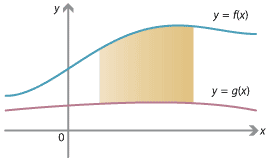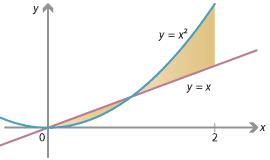## Content

### Area between two curves

So far we have only found areas between the graph $$y=f(x)$$ and the $$x$$-axis. In general we can find the area enclosed between two graphs $$y=f(x)$$ and $$y=g(x)$$. If $$f(x) > g(x)$$, then the desired area is that which is below $$y=f(x)$$ but above $$y=g(x)$$, which is

$\int_a^b \big( f(x) - g(x) \big) \; dx.$

This formula works regardless of whether the graphs are above or below the $$x$$-axis, as long as the graph of $$f(x)$$ is above the graph of $$g(x)$$. (Can you see why?)Detailed description of diagram

In general, two graphs $$y=f(x)$$ and $$y=g(x)$$ may cross. Sometimes $$f(x)$$ may be larger and sometimes $$g(x)$$ may be larger. In order to find the area enclosed by the curves, we can find where $$f(x)$$ is larger, and where $$g(x)$$ is larger, and then take the appropriate integrals of $$f(x) - g(x)$$ or $$g(x) - f(x)$$ respectively.

#### Example

Find the area enclosed between the graphs $$y=x^2$$ and $$y=x$$, between $$x=0$$ and $$x=2$$.Detailed description of diagram

#### Solution

Solving $$x^2 = x$$, we see that the two graphs intersect at $$(0,0)$$ and $$(1,1)$$. In the interval $$[0,1]$$, we have $$x \geq x^2$$, and in the interval $$[1,2]$$, we have $$x^2 \geq x$$. Therefore the desired area is

\begin{align*} \int_0^1 ( x - x^2 ) \; dx \, + \int_1^2 ( x^2 - x ) \; dx &= \Bigg[ \frac{1}{2} x^2 - \frac{1}{3} x^3 \Bigg]_0^1 + \Bigg[ \frac{1}{3} x^3 - \frac{1}{2} x^2 \Bigg]_1^2 \\ &= \Bigg( \Big( \frac{1}{2} - \frac{1}{3} \Big) - \big( 0 \big) \Bigg) + \Bigg( \Big( \frac{8}{3} - 2 \Big) - \Big( \frac{1}{3} - \frac{1}{2} \Big) \Bigg) \\ &= \Big( \frac{1}{6} - 0 \Big) + \Bigg( \frac{2}{3} - \Big( {-} \frac{1}{6} \Big) \Bigg) \\ &= \frac{1}{6} + \frac{5}{6} = 1. \end{align*}

Next page - Links forward - Integration and three dimensions